# Comb Filter Calculations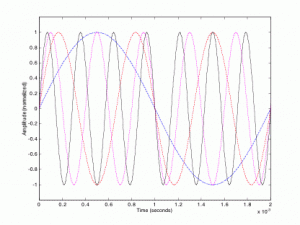You asked for it, so here it is:  the equation that leads to the classic frequency response contour called the comb filter. First, some definitions. We have the undelayed signal

x(t)

and that same signal, delayed by D

x(t-D)

Subtracting D gives the value of the signal D seconds ago.  That delayed signal is likely softer in level, so we include a scaling ‘gain’ g, giving us a neat equation describing the combination of the undelayed plus the delayed signal:

y(t) = x(t) + g*x(t-D)

The equation represents signal plus a scaled, and delayed repetition of that signal. Taking the Fourier Transform and simplifying leads to the Frequency Response:

|H(ω)| = {1 + 2g*cos(ωD) + g^2}^(1/2)

or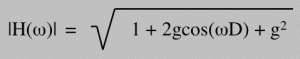ω is frequency in radians. To use Hertz instead, replace ω with 2*pi*ƒ where ƒis frequency in Hertz.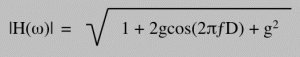Plug that in to your computer or graphing calculator, or bust out some graph paper and dig out your grandfather’s slide rule.  Happy graphing. But don’t forget to give it a listen too. Start with D = 0.001 (that’s 1ms, of course), and sweep the delay time a bit. Presto: the 60s. It looks like this in linear frequency: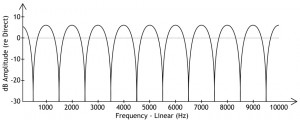Comb Filtering – Linear Frequency

and like this in log frequency: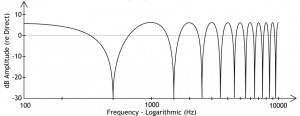Comb Filtering – Logarithmic Frequency